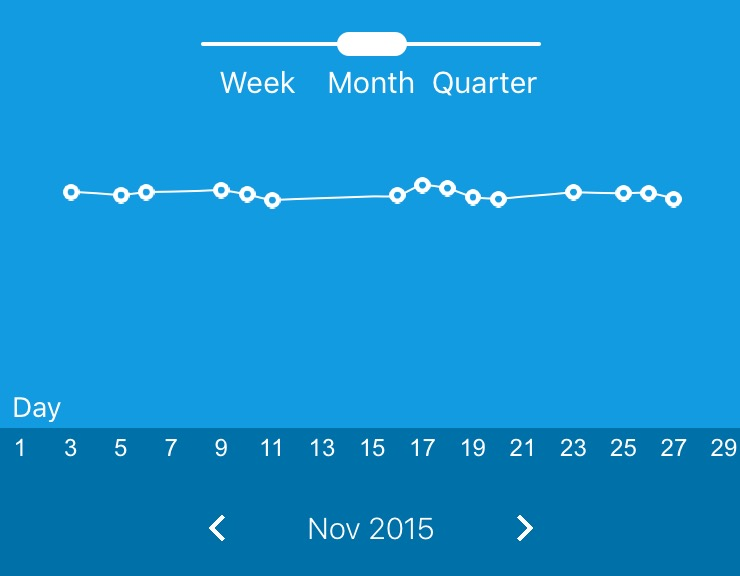# iOS-Echarts------定制性高的图表控件

## 如何使用

• 通过`cocoapods`进行导入：`pod 'iOS-Echarts'`
• 将项目下的`iOS-Echarts`目录拷贝到项目中

### 举个栗子

``````#pragma mark 初始化图表
-(void)initEchartOption {
option = [[PYOption alloc] init];
option.calculable = NO;
option.toolbox = [[PYToolbox alloc] init];
// 坐标轴属性
PYGrid *grid = [[PYGrid alloc] init];
grid.x = @(-2);
grid.y = @(0);
grid.x2 = @(0);
grid.y2 = @(30);
grid.borderWidth = @(0);
option.grid = grid;
// X轴的属性
xAxis = [[PYAxis alloc] init];
xAxis.show = YES;
xAxis.type = @"category";
xAxis.boundaryGap = @(YES);
xAxis.splitLine = [[PYSplitLine alloc] init];
xAxis.splitLine.show = NO;
xAxis.axisTick = [[PYAxisTick alloc] init];
xAxis.axisTick.show = NO;
xAxis.axisLine = [[PYAxisLine alloc] init];
xAxis.axisLine.show = NO;
xAxis.axisLabel = [[PYAxisLabel alloc] init];
xAxis.axisLabel.textStyle = [[PYTextStyle alloc] init];
xAxis.axisLabel.textStyle.color = PYRGBA(255, 255, 255, 1);
option.xAxis = [[NSMutableArray alloc] initWithObjects:xAxis, nil];
// Y轴的属性
yAxis = [[PYAxis alloc] init];
yAxis.type = @"value";
yAxis.show = NO;
yAxis.splitLine = [[PYSplitLine alloc] init];
yAxis.splitLine.show = NO;
yAxis.axisLine.show = NO;
yAxis.axisTick = [[PYAxisTick alloc] init];
yAxis.axisTick.show = NO;
option.yAxis = [[NSMutableArray alloc] initWithObjects:yAxis, nil];
// 折线图的线的属性
NSMutableArray *serieses = [[NSMutableArray alloc] init];
series = [[PYCartesianSeries alloc] init];
series.symbol = @"image://trend_dot@3x.png";
series.symbolSize = @(4);
series.type = @"line";
series.smooth = YES;
series.showAllSymbol = YES;
series.itemStyle = [[PYItemStyle alloc] init];
series.itemStyle.normal = [[PYItemStyleProp alloc] init];
series.itemStyle.normal.lineStyle = [[PYLineStyle alloc] init];
series.itemStyle.normal.lineStyle.color = PYRGBA(255, 255, 255, 1);
series.itemStyle.normal.lineStyle.width = @(1);
int barWidth = [UIScreen mainScreen].bounds.size.width / 10;
series.barWidth = @(barWidth);